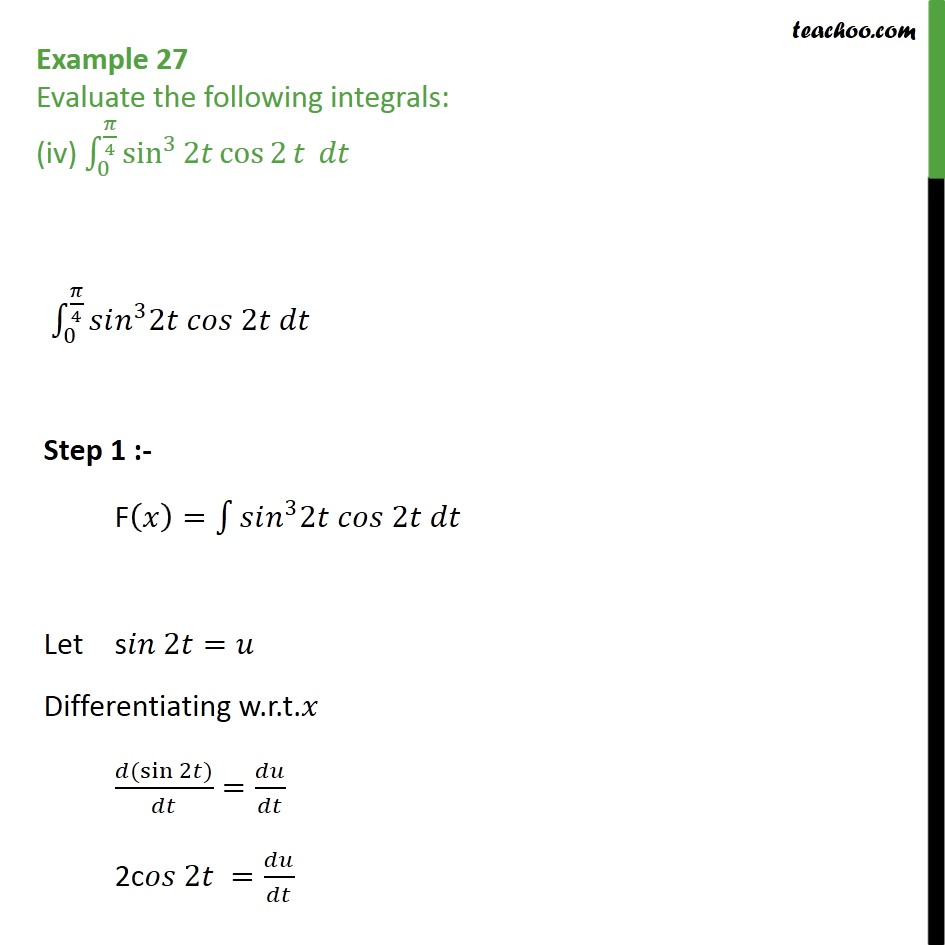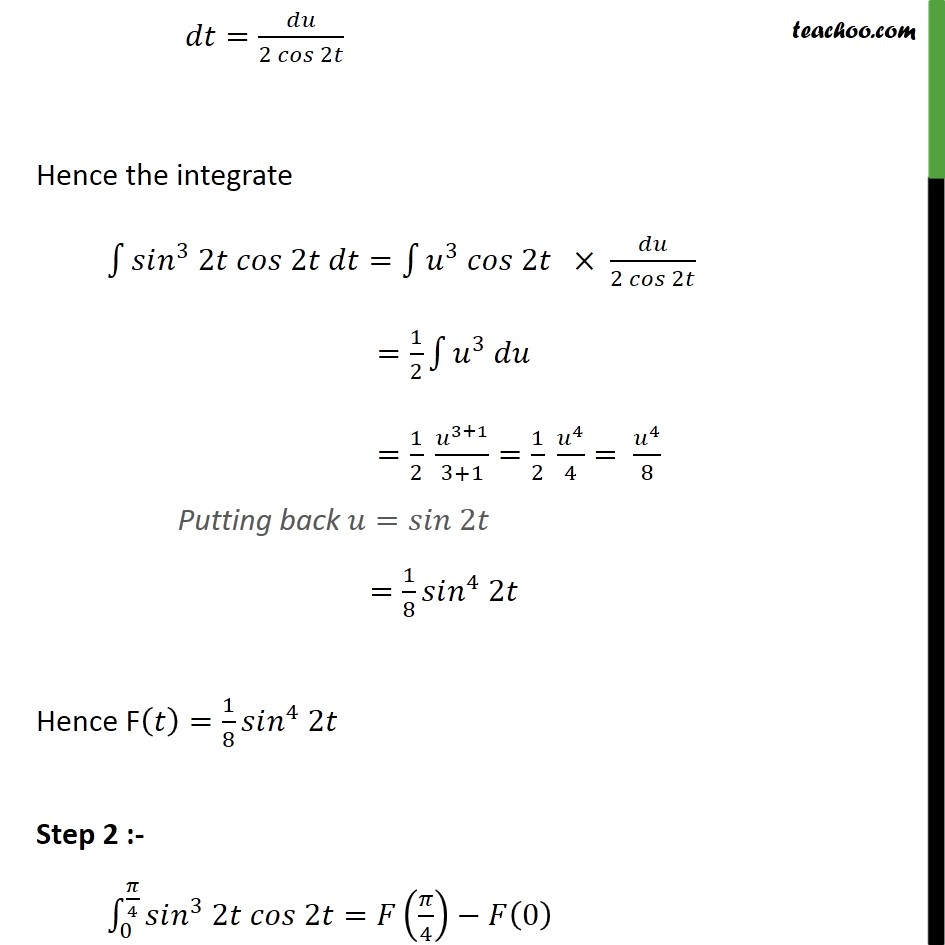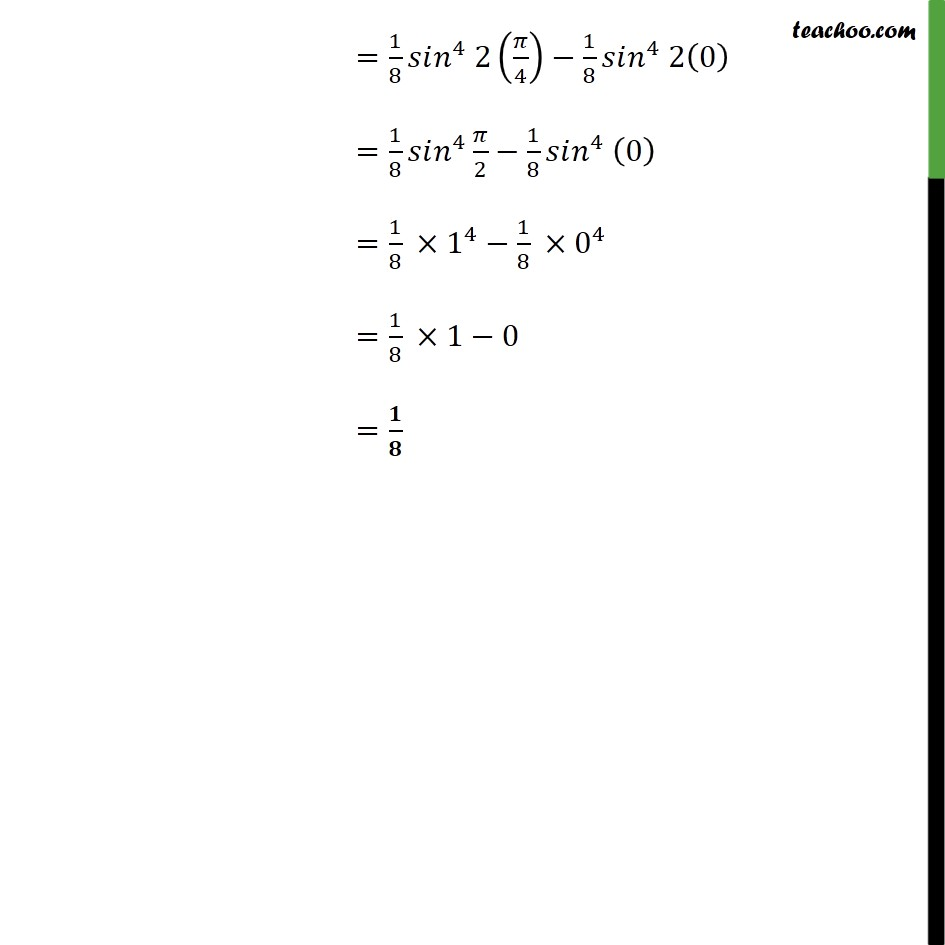Definite Integration - By Substitution

Chapter 7 Class 12 Integrals
Concept wiseLearn in your speed, with individual attention - Teachoo Maths 1-on-1 Class

### Transcript

= Example 27 Evaluate the following integrals: (iv) 0 4 sin 3 2 cos 2 0 4 3 2 2 Step 1 :- F = 3 2 2 Let s 2 = Differentiating w.r.t. ( sin 2 ) = 2c 2 = = 2 2 Hence the integrate 3 2 2 = 3 2 2 2 = 1 2 3 = 1 2 3+1 3+1 = 1 2 4 4 = 4 8 Putting back = 2 = 1 8 4 2 Hence F = 1 8 4 2 Step 2 :- 0 4 3 2 2 = 4 0 = 1 8 4 2 4 1 8 4 2 0 = 1 8 4 2 1 8 4 0 = 1 8 1 4 1 8 0 4 = 1 8 1 0 = 1 8